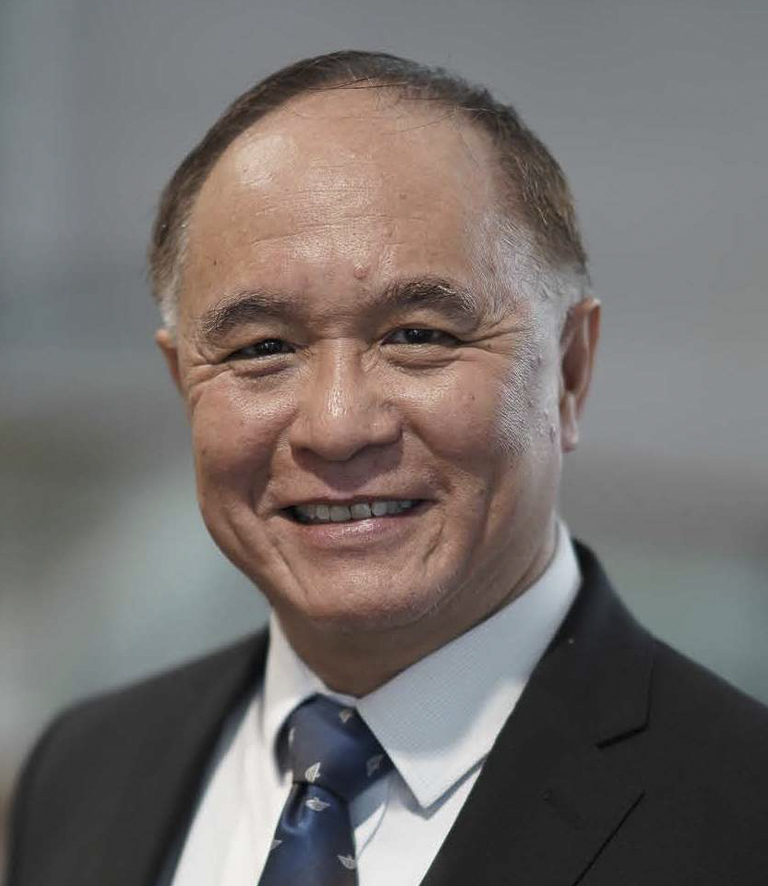Mathematics & Mathematics Education ACADEMIC GROUP### Assoc. Prof. Ang Keng Cheng

Teaching Areas

• Differential Equations and Computational Mathematics
• Master of Science (Mathematics for Educators) Modules on Advanced Calculus and Applied Mathematics
• Inservice Modules on Calculus, Differential Equations and Mathematical Modelling for Secondary and Junior College teachers

Research Interest
His primary interest in research encompasses mathematical modelling in various biological and medical settings (such as blood flow problems, epidemics and tumour growth), as well as numerical and computational methods for partial differential equations. He is also interested in the teaching and learning of mathematical modelling.

Selected Publications
View publications here.